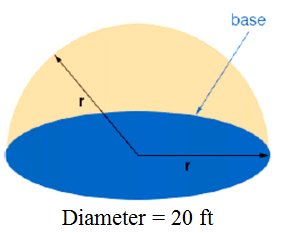# Surface area and volume of spheres - Surface Area and Volume

## What is hemisphere?

Hemispheres are half of spheres. If you took a sphere and cut it exactly in half, you'd have two hemispheres! So for example, a sphere is a ball shape. Cutting it in half will give us two hemispheres of a ball. We'll be working with both spheres and hemispheres in this lesson in order to find surface area and volume.

## How to find the surface area of a sphere

The surface area is the sum of all the surface areas of a shape's sides. In the case of a sphere, the formula for this is:

Surface area = 4$\pi$r^2

## How to find the volume of a sphere

The volume of a sphere tells us how much space there is inside a sphere. How to find volume of a sphere? There's a simple formula for that and it is:

Volume = 4/3 $\pi$r^3

## How to find the surface area of a hemisphere

Let's try out an example that uses the surface area formula for spheres. We're actually not going to deal with a sphere, but a hemisphere.

Question:

Find the following object's surface area:Surface area and volume of spheres

Solution

A hemisphere, as we learned previously, is half of a sphere. Keep this in mind as we work through this problem.

The formula for the surface area of a sphere is:  4$\pi$r^2. To find out the hemisphere's surface area, we can divide this formula by 2 which gives us:  4$\pi$r^2/2. But don't forget that the bottom of the hemisphere now also becomes a surface and will have its own surface area. It's shaped like a circle, and therefore, its surface area is equalled to $\pi$r^2 as we have learned in past lessons. Therefore the formula for our hemisphere is:

4$\pi$r^2/2 + $\pi$r^2

We'll first need to find the radius. We've learnt from previous lessons that the diameter = 2r, so we can make use of this to help us find the radius.

D = 2r

20 = 2r

r = 10 ft

Now we can plug the radius into the formula to solve for the hemisphere's surface area.

Surface area of a hemisphere = 4$\pi$r^2/2 + $\pi$r^2

SA = [4$\pi$(10^2)]2 + $\pi$ (10)^2

SA = 942.48 ft^2

The area of hemisphere equals to 942.48ft^2. Remember that finding surface area will give you answers in squares. Also, do not forget the unit!

## How to find the volume of a hemisphere

Let's move on to finding the volume of a hemisphere.

Question:

Find the following object's volume:Surface area and volume of spheres

Solution:

For the volume, all you'll need to do is to take the formula for a sphere's volume and then divide it by 2. The sphere's formula is Volume = 4/3 $\pi$r^3, and for a hemisphere, we'll use Volume = (4/3 $\pi$r^3)/2.

In terms of calculation we've got:

Volume = (4/3 $\pi$r^3)/2

V = [4/3 $\pi$(10^3)]/2

V = 2094.4ft^3

We get that the volume of hemisphere equals 2094.4ft^3. In this case, since we're finding the volume, the answer will be given in cubic form. Once again, do not forget the unit, which is in feet for this problem.

To see the relationship of the volume and the radius, and how they affect one another, check out this helpful click-and-drag diagram!

### Surface area and volume of spheres

A basketball is a sphere. Soap bubbles are usually spheres. A vitamin capture is a combination of cylinder and sphere. In this lesson, we will learn how to calculate the surface area and volume of spheres and composite solids.

#### Lessons

##### Find the surface area and the volume of the following cones: